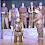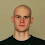## 01 April 2017

### 0.99999999... is equal to 1.000000

This is true.  0.99999.... repeating to infinity is not really really really close to 1.0.  It equals 1.0.
0.9…, or in a variety of other variants such as 0.9, 0.(9)... $0.{\dot$ denotes a real number that can be shown to be the number one. In other words, the symbols "0.999…" and "1" represent the same number. Proofs of this equality have been formulated with varying degrees of mathematical rigor, taking into account preferred development of the real numbers, background assumptions, historical context, and target audience.
There are a variety of ways to demonstrate this to nonbelievers.The most easily understood is to revert to other familiar repeating digits.  Everyone knows that 1/3 is 0.333... and that 2/3 is 0.666...  If you add them together, you get 3/3, which is one.

But now note that the sum of the decimals on the right side of the equation is 0.999...

Therefore, one is equal to (not close to) .999...

You don't agree?  Then try this.  Subtract .999... from one.  What you have is 0.000...  An infinitely long string of zeroes, which can only be equal to zero.  And if the subtraction of .999... from one leaves zero, then the .999... must be one.  But, you say, there's a one at the end that string of zeroes.  No, there isn't, because the string of 9s doesn't end.
Other proofs are offered at the Wikipedia entry.

1.Simplest proof:

x = .999...
10x = 9.999...
10x - x = 9
9x = 9
x = 1

Though the first time you do this (about what, 3rd grade) it _is_ rather freaky.

SMBC had an explanation for this, here:

http://www.smbc-comics.com/comic/monty-hall-problems

Don't forget to press the big red button at the bottom right of the comic for a postscript! :)

Lurker111

1.So you forgot that when you move the decimal point over to the left it leaves one less repeating nine in the product so when you subtract it leaves the remainder that you are missing.
.99999 * 10 = 9.9999
9.99990
- .99999
----------
8.99991

8.99991/9 = .99999

2.The reason this is hard to grasp is that ... is not well defined, except using the mathematical concept of a limit. What it means is, what real number is closest to the infinite series of nines? 1.0 is the closest number. However, any partial sun is always is always less then 1.0.

Lim(1-epsilon)=1 as epsilon goes to zero, but epsilon is never actually zero...

3.To me this has always been perfectly obvious, since 1/3 + 1/3 + 1/3 is obviously one. But a maths teacher of my aquaintance once told me how, as a student, he had once remarked to his teacher that, even though one divided and then multiplied by three is plainly 0.999..., 0.999... is not one. Apparently he expected the entire ediface of mathematics to collapse. The teacher satisfied him with the argument that if A and B are different numbers, you can always find a number in between A and B. And since you can't possibly have a number in between 0.999... and 1, they must be the same thing.

4.I suppose the decimal system is just an approximation of reality.

I also got away with some real lazy math in Physics I class by using ≈ instead of = in most test answers. Learn math kids, it can improve your GPA.

5.2=3 for large values of 2 and small values of 3.

6.I think the reason many people have a hard time with this is that an infinite sum (or anything, for that matter) literally does not make sense until you define what it means. So you have to be very clear about this before you even start. The usual way of defining the value of an infinite sum is as the limit of its partial sums, and the concept of 'limit' also has to be defined clearly. The real argument is then whether those definitions are useful concepts. Of course they are, and for something like 99.999...% of people (pun intended), they are really the only reasonable definitions, even though there are areas of theoretical mathematics (the theory of 'p-adic' numbers) where you would want other definitions. But a conversation about what are the best definitions and why has a different feel than one where you give 5 different 'proofs', all of which obscure the real points, perhaps intentionally.

7.8.Either the number goes on forever or it does not ~
As train rails appear to touch on the horizon, Pi was probably 3 until somebody reached what they thought was their zenith.

9.How many sides does a circle have? One could argue 1 side; readers of Flatland might say very many. I say 2, inside and outside.